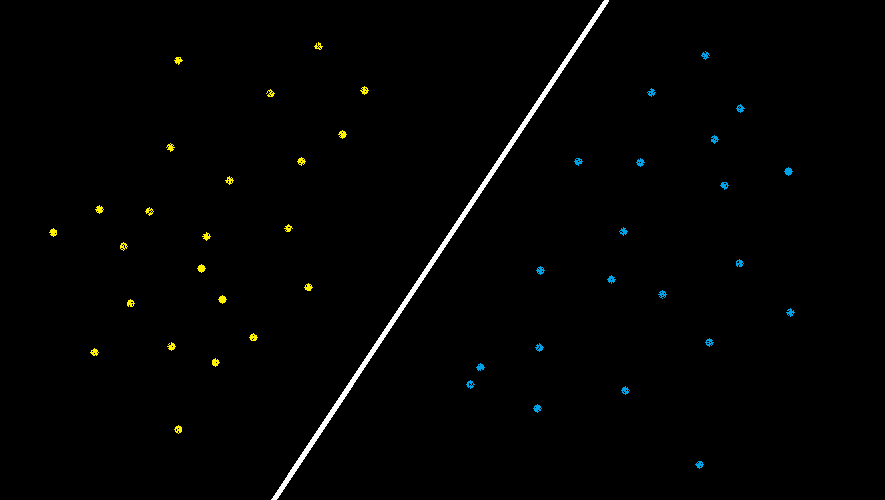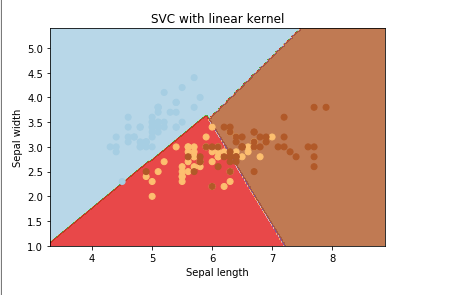Open in App
Not now

# Creating linear kernel SVM in Python

• Difficulty Level : Medium
• Last Updated : 20 Jun, 2018

Prerequisite: SVM

Let’s create a Linear Kernel SVM using the sklearn library of Python and the Iris Dataset that can be found in the dataset library of Python.

Linear Kernel is used when the data is Linearly separable, that is, it can be separated using a single Line. It is one of the most common kernels to be used. It is mostly used when there are a Large number of Features in a particular Data Set. One of the examples where there are a lot of features, is Text Classification, as each alphabet is a new feature. So we mostly use Linear Kernel in Text Classification.In the above image, there are two set of features “Blue” features and the “Yellow” Features. Since these can be easily separated or in other words, they are linearly separable, so the Linear Kernel can be used here.

1. Training a SVM with a Linear Kernel is Faster than with any other Kernel.

2. When training a SVM with a Linear Kernel, only the optimisation of the C Regularisation parameter is required. On the other hand, when training with other kernels, there is a need to optimise the γ parameter which means that performing a grid search will usually take more time.

 `# Import the Libraries``import` `numpy as np``import` `matplotlib.pyplot as plt``from` `sklearn ``import` `svm, datasets`` ` `# Import some Data from the iris Data Set``iris ``=` `datasets.load_iris()`` ` `# Take only the first two features of Data.``# To avoid the slicing, Two-Dim Dataset can be used`` ` `X ``=` `iris.data[:, :``2``]``y ``=` `iris.target`` ` `# C is the SVM regularization parameter``C ``=` `1.0` ` ` `# Create an Instance of SVM and Fit out the data.``# Data is not scaled so as to be able to plot the support vectors``svc ``=` `svm.SVC(kernel ``=``'linear'``, C ``=` `1``).fit(X, y)`` ` `# create a mesh to plot``x_min, x_max ``=` `X[:, ``0``].``min``() ``-` `1``, X[:, ``0``].``max``() ``+` `1``y_min, y_max ``=` `X[:, ``1``].``min``() ``-` `1``, X[:, ``1``].``max``() ``+` `1``h ``=` `(x_max ``/` `x_min)``/``100``xx, yy ``=` `np.meshgrid(np.arange(x_min, x_max, h),``         ``np.arange(y_min, y_max, h))`` ` `# Plot the data for Proper Visual Representation``plt.subplot(``1``, ``1``, ``1``)`` ` `# Predict the result by giving Data to the model``Z ``=` `svc.predict(np.c_[xx.ravel(), yy.ravel()])``Z ``=` `Z.reshape(xx.shape)``plt.contourf(xx, yy, Z, cmap ``=` `plt.cm.Paired, alpha ``=` `0.8``)`` ` `plt.scatter(X[:, ``0``], X[:, ``1``], c ``=` `y, cmap ``=` `plt.cm.Paired)``plt.xlabel(``'Sepal length'``)``plt.ylabel(``'Sepal width'``)``plt.xlim(xx.``min``(), xx.``max``())``plt.title(``'SVC with linear kernel'``)`` ` `# Output the Plot``plt.show()`

Output:Here all the features are separated using simple lines, thus representing the Linear Kernel.

My Personal Notes arrow_drop_up